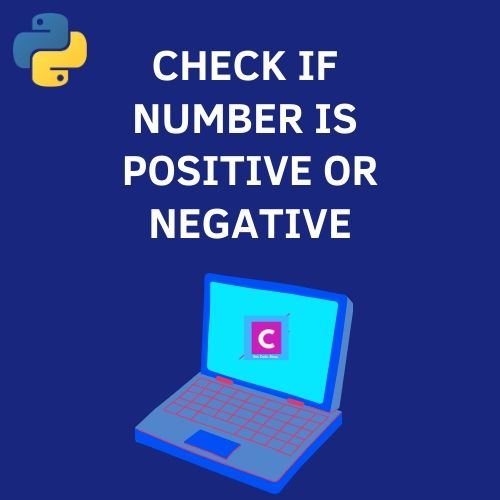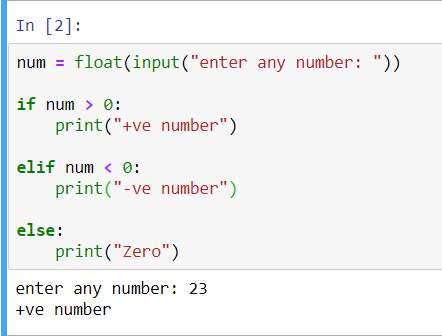# Python 3 Program To Check If Number Is Positive Or Negative

3733In this tutorial, you will find a simple Python 3 program to check if a number is positive or negative. Before moving ahead, you should know about the basics of Python programming language. If you have just started your Python journey, then do check out these best books for beginners to learn Python.

## Python 3 program to check if a number is positive, negative or 0

We can easily do it using Python elif loop where we can check three conditions to see if the number entered by the user is positive or negative.

Condition 1: If the user input is greater than 0, then the number is positive.

Condition 2: If the user input is less than 0, then the number is negative.

Condition 3: If the other two conditions are false, then the number is zero.

Source Code:

```num = float(input("enter any number: "))

if num > 0:
print("+ve number")

elif num < 0:
print("-ve number")

else:
print("Zero")```

## Output